Next: Misunderstandings caused by the Up: Uncertainty in physics and Previous: Probability of the causes   Contents

# Unsuitability of confidence intervals

According to the standard theory of probability, statement () is nonsense, and, in fact, good frequentistic books do not include it. They speak instead about confidence intervals', which have a completely different interpretation [that of ()], although several books and many teachers suggest an interpretation of these intervals as if they were probabilistic statements on the true values, like (). But it seems to me that it is practically impossible, even for those who are fully aware of the frequentistic theory, to avoid misleading conclusions. This opinion is well stated by Howson and Urbach in a paper to Nature:
The statement that such-and-such is a 95% confidence interval for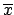seems objective. But what does it say? It may be imagined that a 95% confidence interval corresponds to a 0.95 probability that the unknown parameter lies in the confidence range. But in the classical approach,is not a random variable, and so has no probability. Nevertheless, statisticians regularly say that one can be 95% confident' that the parameter lies in the confidence interval. They never say why.''
The origin of the problem goes directly to the underlying concept of probability. The frequentistic concept of confidence interval is, in fact, a kind of artificial invention to characterize the uncertainty consistently with the frequency-based definition of probability. But, unfortunately - as a matter of fact - this attempt to classify the state of uncertainty (on the true value) trying to avoid the concept of probability of hypotheses produces misinterpretation. People tend to turn arbitrarily () into () with an intuitive reasoning that I like to paraphrase as the dog and the hunter': We know that a dog has a 50% probability of being 100 m from the hunter; if we observe the dog, what can we say about the hunter? The terms of the analogy are clear: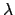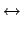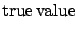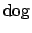The intuitive and reasonable answer is The hunter is, with 50% probability, within 100 m of the position of the dog.'' But it is easy to understand that this conclusion is based on the tacit assumption that 1) the hunter can be anywhere around the dog; 2) the dog has no preferred direction of arrival at the point where we observe him. Any deviation from this simple scheme invalidates the picture on which the inversion of probability ()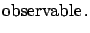() is based. Let us look at some examples.
Example 1:
Measurement at the edge of a physical region.
An experiment, planned to measure the electron-neutrino mass with a resolution of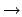(independent of the mass, for simplicity, see Fig.), finds a value of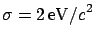(i.e. this value comes out of the analysis of real data treated in exactly the same way as that of simulated data, for which a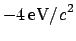resolution was found).
What can we say about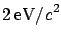?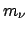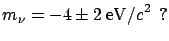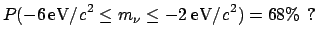No physicist would sign a statement which sounded like he was 98% sure of having found a negative mass!Example 2:
Non-flat distribution of a physical quantity.
Let us take a quantitythat we know, from previous knowledge, to be distributed as in Fig.. It may be, for example, the energy of bremsstrahlung photons or of cosmic rays. We know that an observable value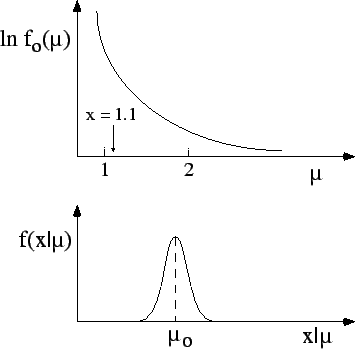will be normally distributed around the true value, independently of the value of. We have performed a measurement and obtained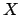, in arbitrary units. What can we say about the true valuethat has caused this observation? Also in this case the formal definition of the confidence interval does not work. Intuitively, we feel that there is more chance thatis on the left side of (1.1) than on the right side. In the jargon of the experimentalists, there are more migrations from left to right than from right to left''.

Example 3:
High-momentum track in a magnetic spectrometer.
The previous examples deviate from the simple dog-hunter picture only because of an asymmetric possible position of the hunter'. The case of a very-high-momentum track in a central detector of a high-energy physics (HEP) experiment involves asymmetric response of a detector for almost straight tracks and non-uniform momentum distribution of charged particles produced in the collisions. Also in this case the simple inversion scheme does not work.

To sum up the last two sections, we can say that intuitive inversion of probability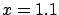(1.5)

besides being theoretically unjustifiable, yields results which are numerically correct only in the case of symmetric problems.Next: Misunderstandings caused by the Up: Uncertainty in physics and Previous: Probability of the causes   Contents
Giulio D'Agostini 2003-05-15